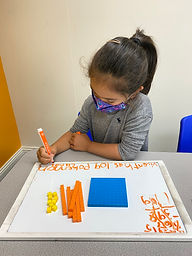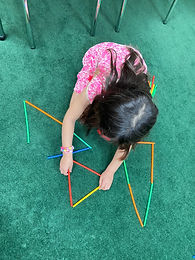## Ms. Brianne

### Target 1​

###### Lesson Type:

New

Number Operation

:

Computation

Solve multi-step word problems within 100 (addition, subtraction, multiplication, and division).

###### 1:

Identify the correct arithmetic processes based on the information presented in word problems.

###### 2:

Find the key words in word problems that indicate the correct arithmetic process to use.

###### 3:

Find and use the needed information in a word problem in order to solve.

3rd

###### Vocabulary:

Activities:

• Students learned what the "CUBES" strategy stands for: C - circle key numbers, U - underline the question, B - box math action words, E - Evaluate what steps to take and eliminate extra information, S - Solve and check.
• Students used the "CUBES" strategy when solving multi-step word problems.### Home Exploration

###### Guiding Questions:## Absent Students:

### Target 2

:

###### 1:

Classify shapes based on side measurements (2D figures).

4th

###### Vocabulary:

Side, Angle, Octagon, Hexagon, Pentagon, Quadrilateral, Parallelogram, Rhombus, Square, Rectangle, Trapezoid, Triangle, Scalene Triangle, Isosceles Triangle, Equilateral Triangle, Right Triangle

Activities:

• Students sorted pictures of 2D shapes by the number of sides or type of shape shown, explaining their reasoning.
• Students sorted various 4-sided shapes by the type of shape (rectangle, square, rhombus, parallelogram, trapezoid).
• Students compared different types of triangles and their characteristics.
• Students were introduced to the names for the different triangles and used straws to create each of them.### Home Exploration

###### Guiding Questions:### Target 3

:

###### Vocabulary:

Activities:### Home Exploration